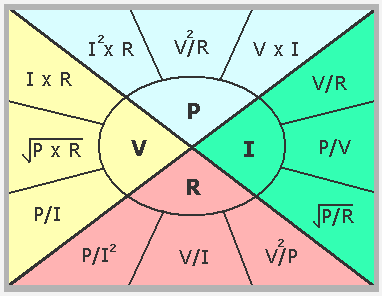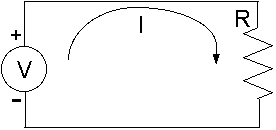### Ohm's LawV = I x R
I = V ÷ R
R = V ÷ I

UNITS
V = Voltage (Volts, V)
I = Current (Amperes, A)
R = Resistance (Ohms, Ω)

### Ohm's Law with Power EquationsV = Voltage (Volts, V)
I = Current (Amperes, A)
R = Resistance (Ohms, Ω)
P = Power (Watts, W)

#### Voltage (V)

Ohm's Law:  V = I x R
In terms of power:  V =  P x R ,  V = P ÷ I

#### Current (I)

Ohm's Law:  I = V ÷ R
In terms of power:  I = P ÷ V,  I =  P ÷ R

#### Resistance (R)

Ohm's Law:  R = V ÷ I
In terms of power:  R = P ÷ I2,  R = V2 ÷ P

#### Power (P)

P = I2 x R,  P = V2 ÷ R,  P = V x I